# Chapter 7 - Coordinate Geometry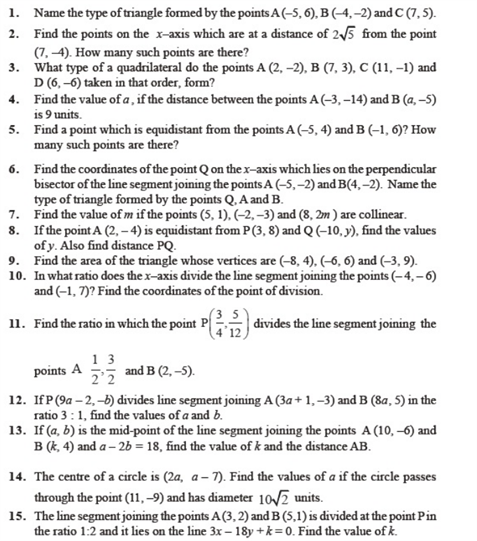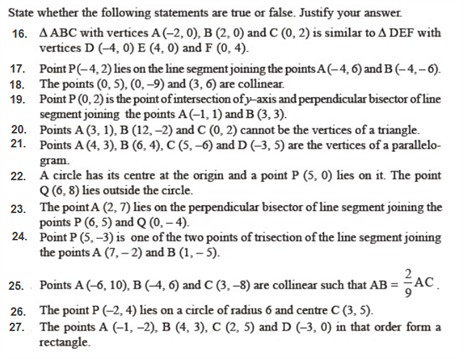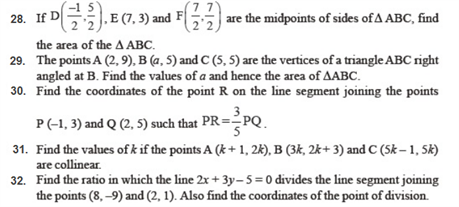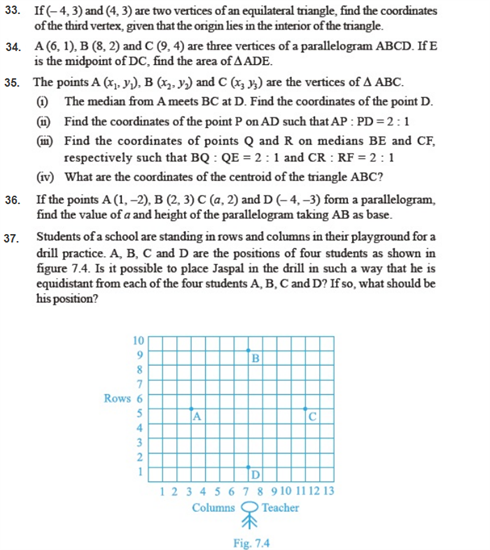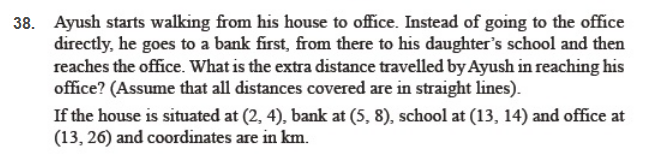#### Practise This Question

Condition satisfied by the pair of equation 2x+3y=5 and 4x+6y10=0 is